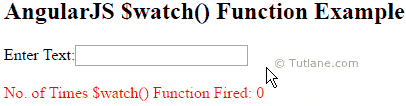# AngularJS \$watch() Function

Here we will learn \$watch() function in angularjs and how to use \$watch() function in angularjs applications to watch scope variable changes with example.

## AngularJS \$watch() Function

In angularjs \$watch() function is used to watch the changes of variables in \$scope object. Generally the \$watch() function will create internally in angularjs to handle variable changes in application.

Suppose in our angularjs applications if we want to create a custom watch for some actions then it’s better to use \$scope.watch function.

To use \$scope.watch() function in angularjs we need to pass two parameters one is expression / function and second one is listener function.

We will see how to use angularjs \$watch() function with expression and function parameters.

## Syntax of AngularJS \$watch() Function

Following is the syntax of using \$watch() function with expression parameter in angularjs applications.

\$scope.\$watch('expression', function (newvalue, oldvalue) {

});

Following is the syntax of using \$watch() function with function parameter in angularjs applications.

\$scope.\$watch(function () {}, function (newvalue, oldvalue) {

});

Now we will see how to use \$watch() function in angularjs applications with example.

## AngularJS \$watch() Function Example

Following is the example of using \$watch() function in angularjs applications.

<!DOCTYPE html>

<html>

<title>

AngularJs \$watch() Function with Example

</title>

<script type="text/javascript">

var app = angular.module('watchApp', []);

app.controller('watchCtrl', function (\$scope) {

\$scope.count = -1;

\$scope.\$watch('txtval', function (newval, oldval) {

\$scope.count = \$scope.count + 1;

});

});

</script>

<body>

<div ng-app="watchApp" ng-controller="watchCtrl">

<h2>AngularJS \$watch() Function Example</h2>

Enter Text:<input type="text" ng-model="txtval" /><br /><br />

<span style="color:Red">No. of Times \$watch() Function Fired: {{count}}</span>

</div>

</body>

</html>

If you observe above code we are counting how many times \$watch() function called in angularjs applications.

## Output of AngularJS \$watch() Function Example

Following is the result of using \$watch() function in angularjs applications.This is how we can use \$watch() function in angularjs applications to watch \$scope variable changes.